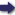(London :  Kegan Paul, Trench, Trübner & Co.,  1910.)

 Tools

## Search this bookPrev Page 29 Next``` CHAPTER LII. 29 six manvantaras and their samdhi, consisting of seven kritayuga, are 676,610,573,760. If we divide this number by 7, we get a remainder of 2. Therefore the six manvantaras end with a Monday, and the seventh begins with a Tuesday. Of the seventh manvantccra there have already elapsed twenty-seven caturyugas, i.e. 42,603,744,150 days. If we divide this number by 7, we get a remainder of 2. Therefore the twenty-eighth caturyuga begins with a Thursday. The days of the yugas which have elapsed of the ■^v&^Qiit caturyuga &YQ 1,420,124,805. The division by 7 gives the remainder i. Therefore the kaliyuga begins with a Friday. Now, returning to our gauge-year, we remark that the years which have elapsed of the kcdp)a up to that year are 1,972,948,132. Multiplying them by 12, we get as the number of their months 23,675,377,584. In the date which we have adopted as gauge-year there is no month, but only complete years; therefore we have nothing to add to this number. By multiplying this number by 30 we get days, viz. 710,261,327,520. As there are no days in the normal date, we have no days to add to this number. If, therefore, we had multiplied the number of years by 360, we should have got the same result, viz. the partial solar days. Multiply this number by 5 311 and divide the pro¬ duct by 172,800. The quotient is the number of the adhimdsa days, viz. 21,829,849,018^-||-. If, in multi¬ plying and dividing, we had used the months, we should have found the adhimdsa months, and, multi¬ plied by 30, they would be equal to the here-mentioned number of adhimdsa days. If we further add the adhimdsa days to the partial solar days, we get the sum of 732,091,176,538, i.e. the partial lunar days. Multiplying them by 55,739, and ```Prev Page 29 Next